# ROBUST MODELING

The median and the mean are two kinds of statistical average. In a normal situation they behave in about the same way. At the present time physical scientists almost always use the mean and hence tend to be unaware of the dramatic ability of the median to cast off the effect of blunders in the data. As an example, consider an expensive, all-day-long experiment which yields only one number for a result. On the first day the result is 2.17, on the second day it is 2.14, and on the third and final day it is 1638.03. The mean of these results is 547.78 but the median (middle value) is 2.17. If one suspects a blunder on the third day, one will obviously prefer the median. Statisticians call this the robust" property of the median. The objective of this section is to show how many kinds of geophysical data fitting can be made to be robust. In particular, all the calculations we now do which amount to solving overdetermined linear simultaneous equations by means of summed squared-error minimization can be made robust by minimizing summed absolute values of errors, instead. Computer costs are often comparable to those of least-squares methods. The algorithms turn out to solve a slightly broader class of problem than minimizing the summed absolute errors. Positive errors may be penalized with a differend weight factor than negative errors. Such an arrangement is called an asymmetric linear norm. A special case of an asymmetric norm is an inequality. Not surprisingly, it turns out that all linear programming problems are special cases of asymmetric linear-norm problems and the solution techniques for asymmetric linear norms are similar to linear programming.

First, we will see why means and medians relate to squares and absolute values. Let xi be an arbitrary number. Let us define m2 by the minimization of the sum of squared differences (called the L2 norm) between m2 and xi: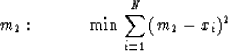(62)

It is a straight forward task to find the minimum by setting the partial derivative of the sum with respect to m2 equal to zero. We get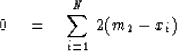or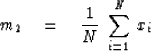(63)

Obviously, m2 has turned out to be given by the usual definition of mean. Next, let us define m1 by minimizing the summed absolute values (called the L1 norm). We have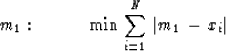(64)

To find the minimum we may again set the partial derivative with respect to m1 equal to zero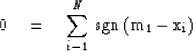(65)

Here the sgn function is +1 when the argument is positive, -1 when the argument is negative, and somewhere in between when the argument is zero. Equation (65) says that m1 should be chosen so that m1 exceeds xi for N/2 terms, m1 is less than xi for N/2 terms, and if there is an xi left in the middle, m1 equals that xi. this defines m1 as a median. [For an even number N the definition (64) requires only the m1 lie anywhere between the middle two values of the xi.]

The computational cost for a mean is proportional to N, the number of points. The cost for completely ordering a list of numbers is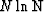,but complete ordering is not required for finding the median. Hoare provided an algorithm for finding the median which requires about 3N operations. A computer algorithm based on Hoare's algorithm will be provided for weighted medians. Weighted medians are analogous to weighted sums. Ordinarily, 2.17 is taken to be the median of the numbers (2.14, 2.17, 1638.03) because we implicitly applied weights (1, 1, 1). If we applied weights (3, 1, 1) it would be like having the numbers 2.14, 2.14, 2.14, 2.17, 1638.03 and the median would then be 2.14. Formally, a weight median may be defined by the minimization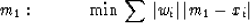(66)

Obviously if the weight factors are all unity, this reduces to the earlier definition whereas using a weight factor equal to 3, for example, is just like including the same term three times with a weight of 1. Figure 2 illustrates the definition (66) for a simple case. From Figure 2 it is apparent that a median is always equal to one of the xi4 even if the weights are not integers. If the weights are all unity and there is an even number of numbers, then the error norm will be flat between the two middle numbers. Then any value in between satisfies our definition of median by minimizing the sum.

 6-3 Figure 2 A sum of weighted absolute value norms. The function labeled A is .5|m-1|, B is .5|m-5|, C is .1|m-2|, and D is the sum of A, B, and C. The sum D is minimized at m-2, a point which exactly solves C = 0 = .1|m-2|.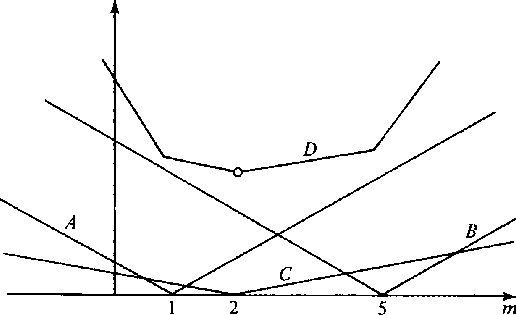Let us rearrange (66) by bringing |wi| into the other absolute-value function. We have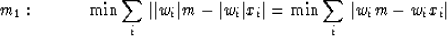(67)

We will now relabel things from the conventions of statistics to the usual convention of simultaneous equations and linear programming. Let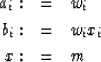(68) (69)
With these new definitions (67) becomes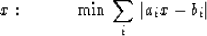(70)
The definition (70) says, in other words, to solve the rank one overdetermined equations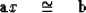(71)
for x by minimizing the L1 norm. This is, in effect, a weighted median problem. If (71) were solved by minimizing the L2 norm (least squares) x would turn out to be the weighted average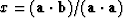.

We now consider a solution technique for the minimization (66). Essentially, it is Hoare's algorithm. On a trial basis we select a random equation from the set (71) to be exactly satisfied. This equation, called the basis equation, can be denoted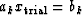.Inserting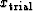into (71) we get equations with positive errors, negative errors, and zero errors. If we have been lucky with,then we find that the zero error group has enough weight to swing the balance between positive and negative weights in either direction. Otherwise, we must pick a new trial basis equation from the stronger of the positive or negative group. Fortunately, we need no longer look into the weaker group because these residuals cannot change signs as we descent into minimum. This may be seen geometrically on a figure like Figure 2. We always wish to go downhill, so once it has been ascertained that a data point is uphill from the present point then it is never necessary to reinspect the uphill point. Thus, the size of the group being inspected rapidly diminishes.

Below are subroutines to compute weighted and skewed medians. (A skewed median" is often called a quantile.) This subroutine is somewhat complicated because it takes special care to do the correct thing when weight factors are zero and because it provides pointers to all equations (occasionally there is more than one) which are satisfied at the final minimum.

subroutine skewer(n,w,f,gu,gd,small,k,t,ml,mh)
# For a Fortran version all in upper case see FGDP p. 126
#       solve rank 1 overdetermined equations with skew norm
#       inputs- n,w,f,gu,gd,small,k.  outputs- k,t,ml,mh.
#   find t to minimize
#        n
#  ls = sum skewnorm(k,f(k)-w(k)*t)
#       k=1
#  where            (gu(k)*(er-small) if er>+small gu>0
#  skewnorm(k,er) = (gd(k)*(er+small) if er<-small gd<0
#                   (0.               if abs(er) <= small
#       gu,gd,w,and f are referenced indirectly as w(k(i)),i=1,n etc
#       minima will be at equations k(ml),k(ml+1),...k(mh).
real w(n),f(n),gu(n),gd(n),g(100)
integer k(n)
integer i,n,low,large,ml,mh,mlt,mht,itry,l
low = 1
large = n
ml = n
mh = 1
gn = 0.
gp = 0.
do itry = 1,n {
l = k(low+mod((large-low)/3+itry,large-low+1))
if (abs(w(l))!=0.) {                # "!=" means "not equal"
t = f(l)/(w(l))
f(l) = w(l)*t
do i = low,large {
l = k(i)
er = f(l)-w(l)*t
g(l) = 0.
if (er>small)
g(l) = -w(l)*gu(l)
if (er<(-small))
g(l) = -w(l)*gd(l)
}
call split(low,large,k,g,mlt,mht)
gnt = gn
do i = low,mlt
gnt = gnt+g(k(i))
gpt = gp
do i = mht,large
gpt = gpt+g(k(i))
gplx = 0.
gmix = 0.
do i = mlt,mht {
l = k(i)
if (w(l)<0.) {
gplx = gplx-w(l)*gu(l)
gmix = gmix-w(l)*gd(l)
}
if (w(l)>0.) {
gplx = gplx-w(l)*gd(l)
gmix = gmix-w(l)*gu(l)
}
}
break 1
low = mht+1
large = mlt-1
if (low>large)
break 1
gn = gnt+gmix
gp = gpt+gplx
ml = mlt
}
mh = mht
}
ml = min0(ml,mlt)
mh = max0(mh,mht)
return
end

subroutine split(low,large,k,g,ml,mh)
# For a Fortran version all in upper case, see FGDP p 127
#	given g(k(i)),i=low,large
#	then rearrange k(i),i=low,large and find ml and mh so that
#	(g(k(i)),i=low,(ml-1)) < 0. and
#	(g(k(i)),i=ml,mh)=0. and
#	(g(k(i)),i=(mh+1),large) > 0.
real g(100)
integer k(100)
#integer low,large,k,ml,mh,keep,i,ii
integer low,large,ml,mh,keep,i,ii
ml = low
mh = large
repeat {
ml = ml-1
repeat
ml = ml+1
until(g(k(ml))>=0)
repeat {
mh = mh+1
repeat
mh = mh-1
until(g(k(mh))<=0)
keep = k(mh)
k(mh) = k(ml)
k(ml) = keep
if (g(k(ml))!=g(k(mh)))
break 1		# Break out of enclosing "repeat{"
do i = ml,mh {
ii = i
if (g(k(i))!=0.0)
go to 30
}
break 2		# Break out of two enclosing "repeat{"'s
30  keep = k(mh)
k(mh) = k(ii)
k(ii) = keep
}
}
return
end


The next step up the ladder of complexity is to consider two unknowns. The obvious generalization of (71) is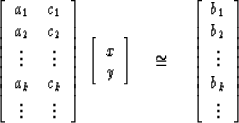(72)
We will assume that the reader is familiar with the solution to (72) by the least-squares method. Solution by minimizing the sum of the absolute values of the errors begins in a similar way. We begin by defining the error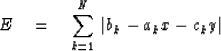(73)
Then we will set the x derivative of the error equal to zero and the y derivative of the error equal to zero.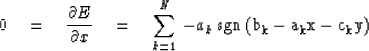(74)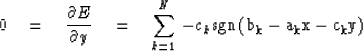(75)
Now we run into a snag. If the sgn function always takes the value +1 or -1, then (74) implies that the ak may be divided into two piles of equal weight. Clearly many, indeed most, collections of numbers cannot be so balanced (for example, if all the ai except one are integers). The difficulty will be avoided if at least one of the equations of (72) is solved exactly so that sgn takes an indeterminate value for the term. Any algebraic confusion may be quickly dispelled by recollection of Figure 2 and the result that even with one unknown the minimum generally occurs at a corner where the first derivative is discontinuous. The same situation must again apply to (75). The usual situation is that for N equations and M unknowns precisely M of the N equations will be exactly satisfied in order to enable the error gradient to vanish at the minimum. Common usage in the field of linear programming is to refer to any nonsingular subset of M out of the N equations as a set of basis equations. The particular set of M equations which is solved when the error is minimized is called the optimum basis.

Although linear programming is a twentieth-century development, the basic ideas seem to have been well known before Laplace in the eighteenth century. Indeed, in the words of Gauss' Theoria Motus Corporum Coelestium which appeared in 1980: Laplace made use of another principle for the solution of linear equations, the number of which is greater than the number of unknown quantities, which had been previously proposed by Boscovich, namely that the differences themselves, both all of them taken positively, should make up as small a sum as possible. It can be easily shown, that a system of values of unknown quantities, derived from this principle alone, must necessarily (except the special cases in which the problem remains, to some extent, indeterminate) exactly satisfy as many equations out of the number proposed, as there are unknown quantities, so that the remaining equations come into consideration only so far as they help to determine the choice.

Next a simple but effective technique for descent down a multidimensional error surface will be described. The position of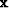on a line through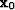can be indicated by a scalar parameter t. The direction of the line can be specified by an M component vector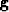.Then any pointon the line may be represented as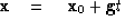(76)
Inserting (76) into the overdetermined set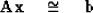(77)
we obtain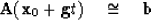(78)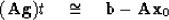(79)
Defining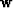andby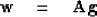(80)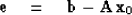(81)
(79) becomes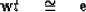(82)
Solving (82) by minimizing the summed absolute errors also given the minimum error along the line in (78). But (82) is the weighted median problem disussed earlier. Recall that the solution t to (82) which gives minimum absolute error will exactly satisfy one of the equations in (82). Let us say t = ek/wk. For this value of t, the kth equation in (77) will also be satisfied exactly. The kth equation in now considered to be a good candidate for the basis, and we will next show how to pick the vectorso as to continue to satisfy the kth equation (stay on the kth hyperplane) as we adjust t in the next iteration.

Now we need a set of basis equations. This is a set of M equations which is temporarily taken to be satisfied. Then, as new equations are introduced into the basis by the weighted median solution, old equations are dropped out. The strategy of the present algorithm is merely to drop out the one which has been in longest. Let us denote our basis equations by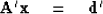(83)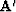is a square matrix. The inverse of the matrixwill be required and will be denoted by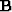.Now suppose we decide to throw out the pth equation from the basis matrix.Then forwe select the pth column of.To see why this works not that since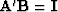the M vector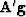will now be the pth column from the identity matrix. Therefore, in the N vector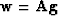there is a component equal to +1, there are M -1 components equal to 0, and there are N - M other unspecified elements. If the kth equation in (77) or (82) has been kept in the basis (83), then the kth equation in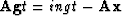now reads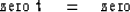(84)
The left-hand zero is an element from the identity matrix and the right-hand zero is from the statement that the kth equation is exactly satisfied. Clearly, we can now adjust t as much as we like to attain a new local minimum and the kth equation will still be exactly satisfied. There is also one equation of the form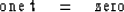(85)

It will be satisfied only if t is zero. Geometrically, this means that if we must move to get to a minimum, then this equation is not satisfied and so we are jumping from this hyperplane. This equation is the one leaving the basis. Of course, if t turns out to be zero, then it reenters the basis. The foregoing steps are iterated until such time that for M successive iterations the quations thrown out of the basis by virtue of its age has immediately reappeared because t = 0. This means that the basis can no longer be improved and we have arrived at the optimum basis and the final solution.